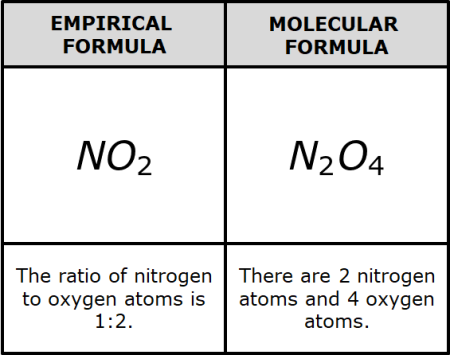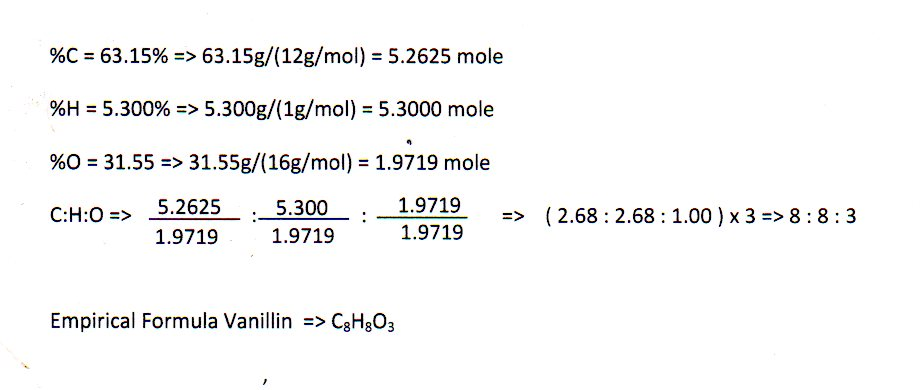Gayathri Mani

# Empirical Formula Study Guide

A formula that presents the components of a compound in its smallest whole-number ratio is known as an empirical formula.

Table of content

## INTRODUCTION

Every now and then, we hear about discovering a new compound (a substance containing atoms of two or more chemical elements held together by chemical bonds). Have you ever wondered how or where these compounds are synthesized or discovered? What difficulties would the scientists have to face in the entire process? The discovery of a new compound represents the beginning of a protracted research endeavor.

We need to know a lot about the composition of this new compound to synthesize it in the lab. Identifying the elements in the content is often a good place to start. The relative quantities of each element may then be determined, allowing us to continue our study of this novel substance. One important part of this research is finding out the empirical formula of the compound. What is an empirical formula? The simplest whole-number ratio of atoms present in a chemical compound is the empirical formula. Let's talk about it in more detail.

### What is Empirical formula?

Ordinarily, empirical formulae are employed to depict compounds with unknown molecular structures or entities that are not made up of regular molecular entities.

To characterize and summarize compounds' atomic components, chemists utilize several notations. The chemical symbols for the elements and quantitative values define atomic composition in these notations, which comprise empirical, molecular, and structural formulations.

The most basic kind of notation is empirical formulas. They have the smallest whole-number ratio of elements in a compound. Unlike molecular formulae, they do not offer information regarding the precise number of atoms in a single substance component. A compound's molecular formula is the same as whole-number multiple empirical formulae.That being said, how do we calculate or establish this empirical formula for any given compound?

### HOW TO CALCULATE THE EMPIRICAL FORMULA

Mass composition data may be used to develop empirical formulae. Combustion analysis, for example, can be utilized in the following ways:

• For a specimen of an unknown organic compound, a carbon-hydrogen and nitrogen analyzer or CHN analysis tool (a device that can measure the composition of a molecule) may be used to determine the statistical fractions of carbon, hydrogen, oxygen, and other elements.
• The relative mass distributions of elements may be translated into moles once the comparative mass components of elements are known.
• The empirical formula is the smallest whole-number ratio of the components that can be calculated.### Empirical Formula Example

Let us look at ribose, with the molecular formula C5H10O5. Because there are two moles of hydrogen for every mole of carbon and oxygen, the empirical formula of ribose becomes the same as the empirical formula of glucose, i.e., CH2O.

Similarly, C6H12O6 is the molecular formula for glucose. This formula contains two moles of hydrogen for each mole of carbon and oxygen. As a result, the empirical formula for glucose is CH2O.

The structural formula of the chemical compound n-hexane is CH3CH2CH2CH2CH2CH3, which indicates that it contains 6 carbon atoms arranged in a chain and 14 hydrogen atoms. Hexane has the molecular formula C6H14 and the empirical formula C3H7, with a C:H ratio of 3:7. Acetic acid's molecular formula is C2H4O2 and formaldehyde's molecular formula is CH2O. They have different molecular formulas but share the same empirical formula, CH2O.

### Using Empirical Formula to Find a Molecular Formula

If you know the molar mass of the compound, you can use the empirical formula to find the molecular formula. Calculate the empirical formula mass and then divide the compound molar mass by the empirical formula mass to accomplish this. This calculates the ratio of the molecular and empirical formulas. To get the subscripts for the molecular formula, multiply all of the subscripts in the empirical formula by this ratio.

### CONCLUSION

• A formula that presents the components of a compound in its least whole-number proportion is known as an empirical formula.
• The empirical formula is indeed the molecular formula if the molecular formula cannot be reduced into a lower whole-number ratio.

### FAQs

1. How do you find the empirical formula?

• In every empirical formula problem, the mass percent of the components in the compound must first be determined.
• Then convert the percentage to grams.
• After that, split all of the masses by their molar masses.
• Choose the smallest mole response and divide all figures by it.

2. Is empirical the simplest form?

The most basic kind of notation are empirical formulas. They have the smallest whole-number ratio of the components in a compound.

3. What is empirical and molecular form?

Molecular formulas display the number of each type of atom in a molecule, and structural formulas reveal how the atoms in a molecule are combined with one another. Empirical formulas demonstrate the basic whole-number ratio of atoms in a compound.

4. What is the empirical formula of hydrogen peroxide?

H2O2 is the empirical formula of hydrogen peroxide.

We hope you enjoyed studying this lesson and learned something cool about Empirical Formula! Join our Discord community to get any questions you may have answered and to engage with other students just like you! Don't forget to download our App to experience our fun, VR classrooms - we promise, it makes studying much more fun! 😎